# Emotion Detection using Bidirectional LSTM

• Last Updated : 30 Sep, 2021

Emotion Detection is one of the hottest topics in research nowadays. Emotion sensing technology can facilitate communication between machines and humans. It will also help to improve the decision-making process. Many Machine Learning Models have been proposed to recognize emotions from the text. But, in this article, our focus is on the Bidirectional LSTM Model. Bidirectional LSTMs in short BiLSTM is an addition to regular LSTMs which is used to enhance the performance of the model on sequence classification problems. BiLSTMs use two LSTMs to train on sequential input. The first LSTM is used on the input sequence as it is. The second LSTM is used on a reversed representation of the input sequence. It helps in supplementing additional context and makes our model fast.

The dataset which we have used is ISEAR (The International Survey on Emotion Antecedents and Reactions). Here, is a glimpse of the dataset.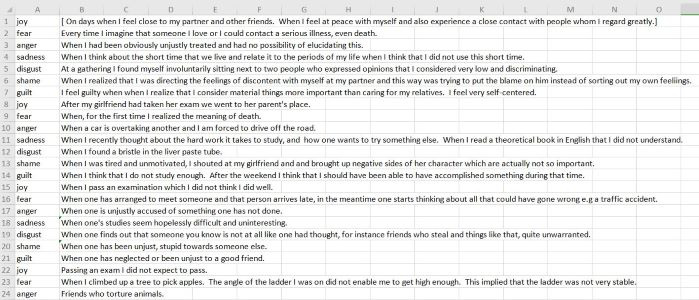ISEAR dataset

ISEAR dataset contains 7652 sentences. It has a total of seven sentiments which are- Joy, Fear, Anger, Sadness, Guilt, Shame, and Disgust.

Let’s go step by step in making the model which will predict emotion.

Step 1: Importing the required libraries

## Python3

 `# Importing the required libraries``import` `keras``import` `numpy as np``from` `keras.models ``import` `Sequential,Model``from` `keras.layers ``import` `Dense,Bidirectional``from` `nltk.tokenize ``import` `word_tokenize,sent_tokenize``from` `keras.layers ``import` `*``from` `sklearn.model_selection ``import` `cross_val_score``import` `nltk``import` `pandas as pd``nltk.download(``'punkt'``)`

Step 2: Next step is to load the dataset from our machine and preprocess it. In the dataset, there are some rows that contain -‘No response’. This sentence is completely useless for us. So, we will drop such rows.

Read the dataset and preprocess it

## Python3

 `df``=``pd.read_csv(``'isear.csv'``,header``=``None``)``# The isear.csv contains rows with value 'No response'``# We need to remove such rows``df.drop(df[df[``1``] ``=``=` `'[ No response.]'``].index, inplace ``=` `True``)`

Step 3:  Apply a word tokenizer to convert each sentence into a list of words. Example: If there is a sentence- ‘I am happy’. Afterward tokenizing it will get converted into a list [‘I’,’am’,’happy’].

Word Tokenize

## Python3

 `# The feel_arr will store all the sentences``# i.e feel_arr is the list of all sentences``feel_arr ``=` `df[``1``]` `# Each  sentence in feel_arr is tokenized by the help of work tokenizer.``# If I have a sentence - 'I am happy'.``# After word tokenizing it will convert into- ['I','am','happy']``feel_arr ``=` `[word_tokenize(sent) ``for` `sent ``in` `feel_arr]``print``(feel_arr[``0``])`

The output of the above code snippet is this: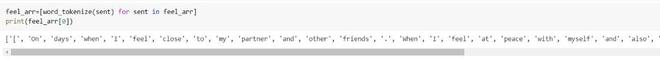The output of word tokenized

Step 4: The length of each sentence is different. To pass it through the model, the length of each sentence should be equal. By visualizing the dataset, we can see that the length of the sentence in the dataset is not greater than 100 words. So, now we will convert every sentence to 100 words. For this, we will take the help of padding.

## Python3

 `# Defined a function padd in which each sentence length is fixed to 100.``# If length is less than 100 , then the word- '' is append``def` `padd(arr):``    ``for` `i ``in` `range``(``100``-``len``(arr)):``        ``arr.append(``''``)``    ``return` `arr[:``100``]``  ` `# call the padd function for each sentence in feel_arr``for` `i ``in` `range``(``len``(feel_arr)):``  ``feel_arr[i]``=``padd(feel_arr[i])`

The output of the above code snippet is this: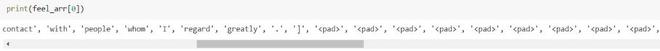5.  Now, each word needs to be embedded in some numeric representation, as the model understands only numeric digits. So, for this, we have downloaded a predefined glove vector of 50 dimensions from the internet. This vector is used for the purpose of word embedding. Each word is represented into a vector of 50 dimensions.

The glove vector contains almost all words of the English dictionary.

Here’s some insight into the glove vector.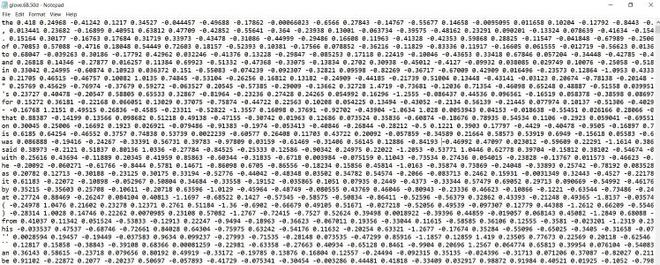Glove vector

The first word of each row is the character that is to be embedded. And from the column to the last column, there is the numeric representation of that character in a 50d vector form.

Word embedding using the glove

## Python3

 `# Glove vector contains a 50 dimensional vector corresponding to each word in dictionary.``vocab_f ``=` `'glove.6B.50d.txt'` `# embeddings_index is a dictionary which contains the mapping of``# word with its corresponding 50d vector.``embeddings_index ``=` `{}``with ``open``(vocab_f, encoding``=``'utf8'``) as f:``    ``for` `line ``in` `f:``        ``# splitting each line of the glove.6B.50d in a list of items- in which``        ``# the first element is the word to be embedded, and from second``        ``# to the end of line contains the 50d vector.``        ``values ``=` `line.rstrip().rsplit(``' '``)``        ``word ``=` `values[``0``]``        ``coefs ``=` `np.asarray(values[``1``:], dtype``=``'float32'``)``        ``embeddings_index[word] ``=` `coefs``        ` `        ` `# Now, each word of the dataset should be embedded in 50d vector with``# the help of the dictionary form above.` `# Embedding each word of the feel_arr``embedded_feel_arr ``=` `[]``for` `each_sentence ``in` `feel_arr:``    ``embedded_feel_arr.append([])``    ``for` `word ``in` `each_sentence:``        ``if` `word.lower() ``in` `embeddings_index:``            ``embedded_feel_arr[``-``1``].append(embeddings_index[word.lower()])``        ``else``:``                ``# if the word to be embedded is '' append 0 fifty times``            ``embedded_feel_arr[``-``1``].append([``0``]``*``50``)`

Here, in the above example, the dictionary formed i.e embeddings_index contains the word and its corresponding 50d vector, to visualize it let’s print the 50 dimensions of the word -‘happy’.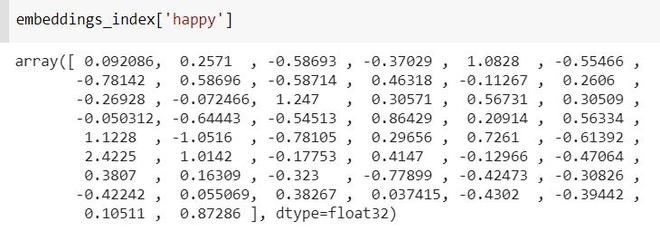Step 6: Now, we are done with all the preprocessing part, and now we need to perform the following things:

• Do one-hot encoding of each emotion.
• Split the dataset into train and test sets.
• Train the model on our dataset.
• Test the model on the test set.

Training the Model

## Python3

 `#Converting x into numpy-array``X``=``np.array(embedded_feel_arr)``print``(np.shape(X))` `# Perform one-hot encoding on df i.e emotion``from` `sklearn.preprocessing ``import` `OneHotEncoder``enc ``=` `OneHotEncoder(handle_unknown``=``'ignore'``)``Y ``=` `enc.fit_transform(np.array(df[``0``]).reshape(``-``1``,``1``)).toarray()` `# Split into train and test``from` `keras.layers ``import` `Embedding``from` `sklearn.model_selection ``import` `train_test_split``X_train, X_test, Y_train, Y_test ``=` `train_test_split(X, Y, test_size``=``0.2``, random_state``=``42``)` `#Defining the BiLSTM Model``def` `model(X,Y,input_size1,input_size2,output_size):``  ``m``=``Sequential()``  ` `  ``# Here 100 denotes the dimensionality of output spaces.``  ` `  ``m.add(Bidirectional(LSTM(``100``,input_shape``=``(input_size1,input_size2))))``  ``m.add(Dropout(``0.5``))``  ``m.add(Dense(output_size,activation``=``'softmax'``))``  ``m.``compile``(``'Adam'``,``'categorical_crossentropy'``,[``'accuracy'``])``  ``m.fit(X,Y,epochs``=``32``, batch_size``=``128``)``  ``return` `m`

Training the model

## Python3

 `# Training the model``bilstmModel``=``model(X_train,Y_train,``100``,``50``,``7``)`

This is the diagram of the proposed model :

Here, the dimension of input is 100 X 50 where 100 is the number of words in each input sentence of the dataset and 50 represents the mapping of each word in a 50d vector.

The output of Bidirectional(LSTM) is 200 because above we have defined dimensionality of output space to be 100. As it a BiLSTM model, so dimensionality will be 100*2 =200, as a BiLSTM contains two LSTMs layers- one forward and the other backward.

After this dropout layer is added to prevent overfitting. And at last dense layer is applied to convert the 200 output sequences to 7, as we have only 7 emotions, so the output should be of seven dimensions only.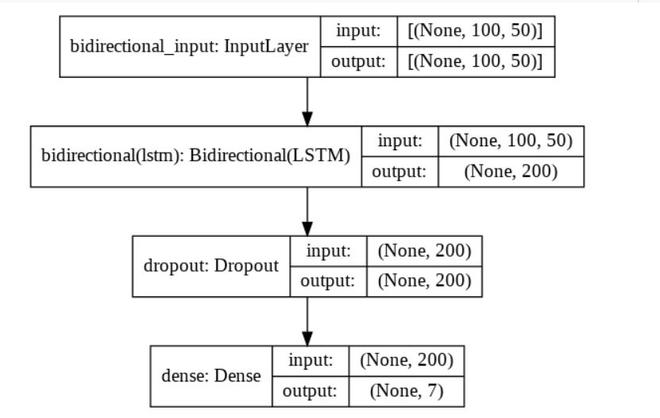Proposed BiLSTM model

Testing the model

## Python3

 `#Testing the model``bilstmModel.evaluate(X_test,Y_test)`

This is the accuracy when we test the model.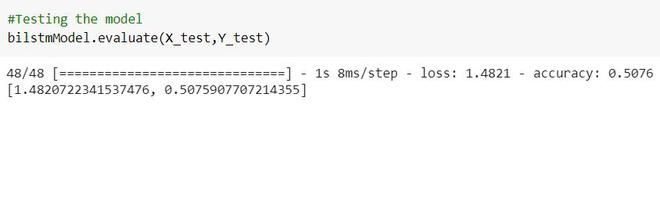Testing accuracy

To get the dataset and code, click here.

My Personal Notes arrow_drop_up